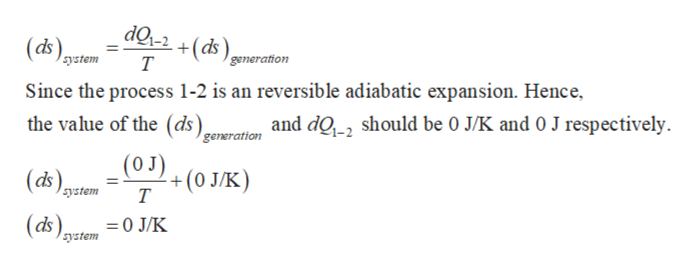# Carbon Oxide (CO) initially occupying 1.8 m3 at 5.2 bar, 246.85°C undergoes an internally reversible expansion during whichpV1.4 = constant to a final state where the temperature is 36.85°C. Assuming the ideal gas model, determine the entropy change, in Joules/K.

Question
8 views

Carbon Oxide (CO) initially occupying 1.8 m3 at 5.2 bar, 246.85°C undergoes an internally reversible expansion during whichpV1.4 = constant to a final state where the temperature is 36.85°C. Assuming the ideal gas model, determine the entropy change, in Joules/K.

check_circle

Step 1

In the problem it is provided that the CO follow the reversible expansion process pv1.4=constant. Since the carbon monoxide is a diatomic gas, the value of adiabatic index for CO is 1.4. Thus, the above process is to be considered as a reversible adiabatic expansion.

Step 2

The expression for change in entropy of t...help_outlineImage TranscriptionclosedQi-2 +(ds т (assystem generation Since the process 1-2 is an reversible adiabatic expansion. Hence, and dQ2 should be 0 J/K and 0 J respectively the value of the (ds) generation (O J) (ds eystem +(0 JK) Т (ds 0 J/K system fullscreen

### Want to see the full answer?

See Solution

#### Want to see this answer and more?

Solutions are written by subject experts who are available 24/7. Questions are typically answered within 1 hour.*

See Solution
*Response times may vary by subject and question.
Tagged in

### Thermodynamics